# Python 命名空间和范围 (作用域）

## Python 中的名称是什么？

``````

# Note: You may get different values for the id

a = 2
print('id(2) =', id(2))

print('id(a) =', id(a))``````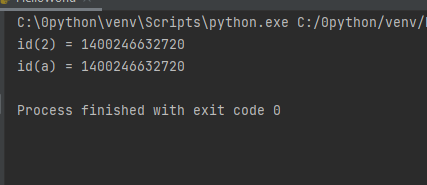``````# Note: You may get different values for the id

a = 2
print('id(a) =', id(a))

a = a+1
print('id(a) =', id(a))

print('id(3) =', id(3))

b = 2
print('id(b) =', id(b))
print('id(2) =', id(2))``````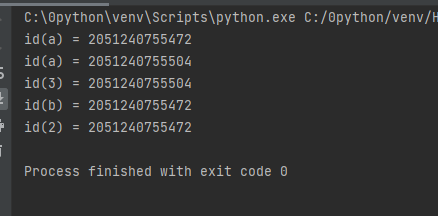``````>>> a = 5
>>> a = 'Hello World!'
>>> a = [1,2,3]``````

``````def printHello():
print("Hello")

a = printHello

a()``````

``Hello``

`a`可以使用相同的名称，我们可以使用该名称来调用该函数。

## 2. Python 中的命名空间是什么？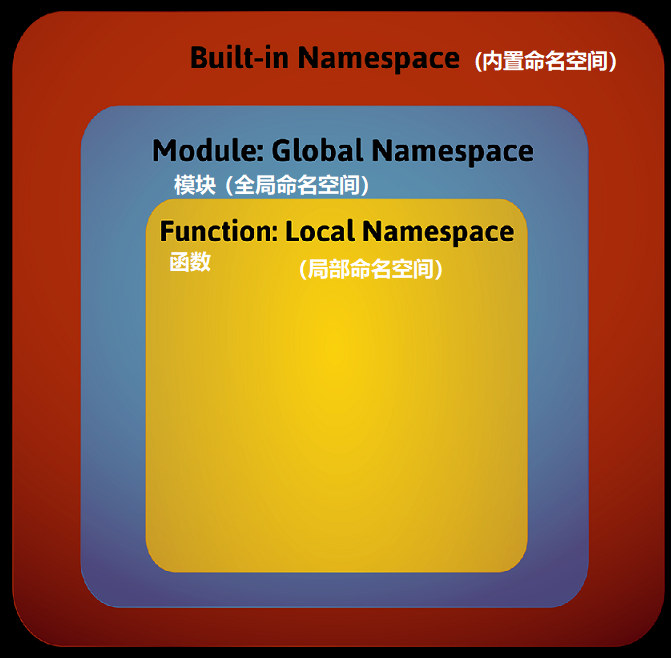## 3.Python 变量范围

1. 具有局部名称的当前函数的范围
2. 具有全局名称的模块的范围
3. 具有内置名称的最外部作用域

## 4.Python 范围(作用域）和命名空间的示例

```def outer_function():
a = 20

def inner_function():
a = 30
print('a =', a)

inner_function()
print('a =', a)

a = 10
outer_function()
print('a =', a)
```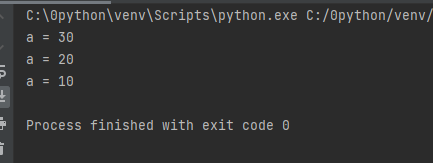```def outer_function():
global a
a = 20

def inner_function():
global a
a = 30
print('a =', a)

inner_function()
print('a =', a)

a = 10
outer_function()
print('a =', a)
```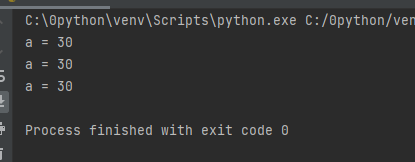wer

(Visited 43 times, 1 visits today)

Posted in Python教程